# Trigonometric sum

(diff) ← Older revision | Latest revision (diff) | Newer revision → (diff)

A finite sumof the form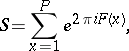where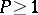is an integer andis a real-valued function of. More general sumsof the following form are also called trigonometric sums: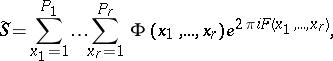whereis a real-valued function andis an arbitrary complex-valued function.

Ifis a polynomial, thenis called a Weyl sum; if the polynomialhas rational coefficients,thenis called a rational trigonometric sum; if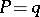, then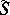is called a complete trigonometric sum; ifand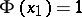when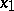is a prime number while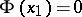whenis a composite number, thenis called a trigonometric sum over prime numbers; if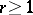,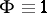andis a polynomial, thenis called a multiple Weyl sum. A basic problem in the theory of trigonometric sums is that of finding upper bounds for the moduli ofand.

How to Cite This Entry:
Trigonometric sum. Encyclopedia of Mathematics. URL: http://encyclopediaofmath.org/index.php?title=Trigonometric_sum&oldid=15501
This article was adapted from an original article by A.A. Karatsuba (originator), which appeared in Encyclopedia of Mathematics - ISBN 1402006098. See original article